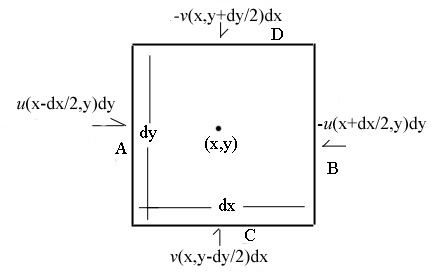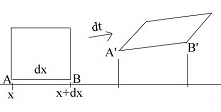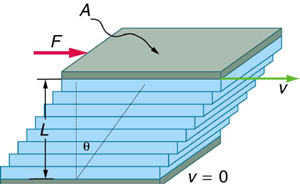# Calculus Without Tears

### Outline of the derivation for the momentum equations

The 2-D Navier-Stokes equations consist of an x-momentum equation, a y-momentum equation, and a continuity equation. The momentum equations are derived from Newton's Second Law, F = MA which is rewritten as a momentum equation MV' = F. Newton's law applies to objects and we want to apply it to a fixed control volume in a moving fluid, that is, fluid is continually flowing in and out of the control volume. As a 'particle' of fluid enters the control volume its momentum is added to that of the control volume, and as a 'particle' of fluid flows out, its momentum is subtracted from that of the control volume, Reynold's Transport Theorem states that this momentum is added to the momentum in the control volume, so it affects the rate of change of momentum in the control volume. So that

MV' for the control volume = F + (momentum flowing in - momentum flowing out)

Fluid is flowing into the control volume thru the left side at the rate ρ·u(x-dx,y)·dy where ρ is the fluid density. Thus, the flow rate of x-momentum into the control volume thru the left side is
u·ρ·u(x-dx,y)·dy.After a few steps (in the book) the per unit volume flow rate of x-momentum into the control volume thru the left and right sides total is shown to be
-ρ·u·du/dx
and the flow rate of x-momentum into the control volume thru the top and bottom sides total is
-ρ·u·dv/dy.

The per unit volume rate of change of x-momentum of the fluid in the control volume is ρ·du/dx and so
ρ·du/dx = -ρ·u·du/dx - ρ·u·dv/dy + F
which is rewritten
ρ·du/dx + ρ·u·du/dx + ρ·u·dv/dy = F
and we have the left side of the equation.

The right side of the x-momentum equation is Fx. The forces acting on the control volume are divided into the force due to pressure P and the force due to fluid motion. To calulate the forces on the control volume we need to calculate the stresses on each side of the control volume. The stresses are due to forces due to pressure and to fluid motion.

We need to find expressions for strain rates in terms of the velocity variables, and then to find the relationship between stress and strain rates.

For elastic objects, stress is inferred from strain. For moving fluids stress does not create displacements, it creates acceleration of displacements. Consider the displaced control volume after time dt as shown in the diagram.The strain along the bottom edge at time dt is (u(x+dx,y)-u(x,y))·dt/dx, letting dx -> 0, the strain is ∂u/∂x·dt and the strain rate is ∂u/∂x. Thus for normal strains we have
xx/dt=∂u/∂x
yy/dt=∂v/∂y

Let σ-- be the stresses acting on the control volume. The stresses are partitioned into the stress caused by pressure P, and stress caused by fluid motion τ--. Then the x-force on the left side of the control volume is given by
P(x,y)·dy - τxxdy - τyx·dx,
and the x-force per unit volume is given by
-dP/dx + dτxx/dx + dτyx/dy

To evaluate these force/volume expressions we need to write the stresses due to fluid motion τ-- in terms of velocity gradients. So we relate stress to strain rates and strain rates to velocity gradients. Everything follows from Newton's Law of Viscosity, which states that shear stress in a fluid is proportional to the shear strain rate, and the right assumptions.

Consider fluid between two extensive plates, with the bottom plate stationary and the top plate moving to the right with velocity v. The motion of the top plate will cause the fluid immediately below it to move with velocity v, matching the speed of the plate,and that fluid will in turn cause the fluid below it to move at a slower speed, and so on, so that the fluid between the plates will be moving to the right, and the velocity of the fluid will be function of its distance from the bottom plate.

Newton’s Law of Viscosity states that the change in velocity is linear between the plates, and that the force required to keep the plate moving with velocity v is given by
τ = F / A = μ (v/L) where τ = shear stress and μ = the viscosity coefficient of the fluid.

The shear strain rate is dθ/dt, and in the diagram dθ/dt = du/dy. Generally
dθ/dt = du/dy + dv/dx.
From the diagram θ=v·dt/L
so
τxy = F / A = μ·(v/L) = μ·(du/dy + dv/dx)
and we have the relationship between shear stress and the velocity function.

The relationship between normal stresses and normal strain rates follows from the assumptions that the fluid is incompressible, hence the continuity equation, and that the fluid is isotropic. This is a bit of a sticky wicket, and three derivations are given in the book, the result of all that huffing and puffing is
τxx = 2·μ·du/dx
τyy = 2·μ·dv/dy

With the given expressions for the stresses due to fluid motion in terms of velocity gradients, the derivation of the last term in the momentum equation is straightforward.